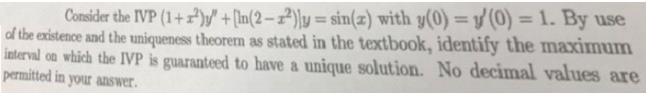Consider the IVP (1+) +[In(2-2)y = sin(z) with y(0) = y(0) = 1. By useof the existence and the uniqueness theorem as stated in the textbook, identify the maximuminterval on which the IVP is guaranteed to have a unique solution. No decimal values arepermitted in your answer.

Questionhelp_outlineImage TranscriptioncloseConsider the IVP (1+) +[In(2-2)y = sin(z) with y(0) = y(0) = 1. By use of the existence and the uniqueness theorem as stated in the textbook, identify the maximum interval on which the IVP is guaranteed to have a unique solution. No decimal values are permitted in your answer. fullscreen
Step 1

To determine the maximum range of values of x for which th...

Want to see the full answer?

See Solution

Want to see this answer and more?

Our solutions are written by experts, many with advanced degrees, and available 24/7

See Solution
Tagged in

Math